• 区域生长法
2021-04-21 08:28:53

本帖最后由 ZoeCiel 于 2015-4-9 16:29 编辑

function Untitled_31_Callback(hObject, eventdata, handles)

% hObject    handle to Untitled_31 (see GCBO)

% eventdata  reserved - to be defined in a future version of MATLAB

% handles    structure with handles and user data (see GUIDATA)

I=handles.img;

axes(handles.axes2);

I=rgb2gray(I);

I=double(I);

[M,N]=size(I);

[y,x]=getpts;          %获得区域生长起始点

x1=round(x);          %横坐标取整

y1=round(y);          %纵坐标取整

seed=I(x1,y1);           %将生长起始点灰度值存入seed中

Y=zeros(M,N);       %作一个全零与原图像等大的图像矩阵Y，作为输出图像矩阵

Y(x1,y1)=1;          %将Y中与所取点相对应位置的点设置为白场

sum=seed;              %储存符合区域生长条件的点的灰度值的和

suit=1;                 %储存符合区域生长条件的点的个数

count=1;             %记录每次判断一点周围八点符合条件的新点的数目

threshold=15;    %域值

while count>0

s=0;                %记录判断一点周围八点时，符合条件的新点的灰度值之和

count=0;

for i=1:M

for j=1:N

if Y(i,j)==1

if (i-1)>0 && (i+1)0 && (j+1)

for u= -1:1                            %判断点周围八点是否符合域值条件

for v= -1:1                            %u,v为偏移量

if   Y(i+u,j+v)==0 & abs(I(i+u,j+v)-seed)<=threshold& 1/(1+1/15*abs(I(i+u,j+v)-seed))>0.8%判断是否未存在于输出矩阵Y，并且为符合域值条件的点

Y(i+u,j+v)=1;                       %符合以上两条件即将其在Y中与之位置对应的点设置为白场

count=count+1;

s=s+I(i+u,j+v);                   %此点的灰度之加入s中

end

end

end

end

end

end

end

suit=suit+count;                                   %将n加入符合点数计数器中

sum=sum+s;                                  %将s加入符合点的灰度值总合中

seed=sum/suit;                                  %计算新的灰度平均值

end

imshow(Y);

handles.img=Y;

guidata(hObject,handles);

就是这段程序  单独在matlab命令行里可以运行  但是一放到GUI的回调函数中  总是显示错误

“  下标索引必须为正整数类型或逻辑类型。

出错 untitled1>Untitled_31_Callback (line 171)

seed=I(x1,y1);           %将生长起始点灰度值存入seed中 ”

应该怎么改呢2015-4-9 16:27 上传

点击文件名下载附件

47.32 KB, 下载次数: 385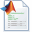2015-4-9 16:27 上传

点击文件名下载附件

21.29 KB, 下载次数: 401

更多相关内容
• 提出了基于种子生长法的图像分割，选取最大值为种子，确定4/8邻域，相似性准则
• 基于区域生长法的图像分割matlab代码明显的结果 该存储库中的代码是Shih和Cheng撰写的论文“用于彩色图像分割的自动播种区域生长”的MATLAB实现。 该方法包括4个主要部分： 将RGB图像转换为YCbCr颜色空间 自动选种 ...
• 区域生长法分割matlab代码球体成像 项目 6 - 多细胞肿瘤球体的分割 Baptiste Alberti - Tanguy Pace - Titouan Poquillon - Pierrick Ullius 文件的组织 代码文件夹 该文件夹包含所有 Matlab 代码文件和 Ilastik ...
• 基于区域生长法的图像分割matlab代码 DIPBigHW 下载此代码 命令行下载 git clone https://github.com/githubxiaowei/DIPBigHW.git 或者如图直接点击下载： 数据集下载 数 据 集 下 载 链 接 （ 图 像 + 所 有 标 注 ...
• 基于区域生长法的图像分割matlab代码这些代码在“磁化传递加权EPI有助于原生fMRI空间中皮层深度的确定”一文中使用。 它使用了公开可用的软件包，包括AFNI，SPM，ANT，FreeSurfer和LAYNII。 数据位于 作者：柴玉辉，...
• 区域生长法分割matlab代码执行摘要 该项目旨在自动化 CNT TEM 图像的直径测量。 目前，它可以区分包含适合测量的 CNT 的感兴趣区域和不包含的感兴趣区域。 这是实现整个过程自动化的第一个障碍。 问题陈述 研究人员...
• 区域生长算法的设计主要由以下三点：生长种子点的确定，区域生长的条件，区域生长停止的条件。 代码采用交互的方式，用鼠标选取合适的种子点，搜索种子点8邻域内的像素。MATLAB
• 利用区域生长法来实现图像分割，本人已经用肝部CT图像来测试过，非常好用。
• 用visual studio2015编译实现的区域生长法去实现图像分割。注意opencv的环境配置。
• 区域生长法MATLAB版本程序代码，需要把图像名字改成程序里一致的 区域生长法MATLAB版本程序代码，需要把图像名字改成程序里一致的图像分割
• 由于图像的复杂性，使得我们无法用阈值化分割时，区域生长法有时候是一个种不错选择，本文介绍了二种算法的综合运用
• 区域生长算法分割图像。共两个函数，一个是以迭代为原理，另一个则以堆为原理。
• 本程序主要功能为实现基于区域生长法的图像分割，选取种子点，并将灰度差值小于阈值的像素点进行合并生长。
• 基于vs2010与opencv采用区域生长法实现的图像分割法，经调试可用，一个挺简单的源程序，可供初学者参考
• 基于区域生长法的图像分割，MATLAB语言，配有程序解释说明
• 本算法包括区域生长法和形态学后处理算法，功能实现了皮肤镜图像的病变区域分割，准确度很高，后处理算法对原图的影响很小；
• 适合二值图像，通过自动扫描提取种子点，注释详细 参考文献：一种二值图像连通区域标记的新方法 陈柏生著。
• 图像分割的两种有效方法，阈值分割和区域生长。其中，阈值分割适合目标区域与其背景在灰度特性上的差异比较大情况。另外，效果较好的区域生长法可以为大家提供参考
• 基于区域生长法的图像分割matlab代码活细胞成像中神经元的高通量检测和跟踪 Neuritetracker是用于在活细胞成像中对神经元或神经元样细胞进行高通量检测，跟踪和分段的软件。 它分析图像序列，并将结果存储为电影和...
• 该程序用于实现图像分割中的区域生长法。根据灰度差和与种子点是否相邻判断是否生长
• 很全面的图像分割matlab代码，内含分水岭算法，区域生长法等，亲试，很好用
• 本程序主要功能为实现基于区域生长法的图像分割，选取种子点，并将灰度差值小于阈值的像素点进行合并生长。
• 传统的区域分割方法有区域生长和区域分裂与合并，其中最基础的是区域生长法区域生长法 区域生长是根据事先定义的准则将像素或者子区域聚合成更大区域的过程。其基本思想是从一组生长点开始（生长点可以是单个像素...

我们将讨论以区域为基础的图像分割处理技术。传统的区域分割方法有区域生长和区域分裂与合并，其中最基础的是区域生长法。

区域生长法

区域生长是根据事先定义的准则将像素或者子区域聚合成更大区域的过程。其基本思想是从一组生长点开始（生长点可以是单个像素，也可以是某个小区域），将与该生长点性质相似的相邻像素或者区域与生长点合并，形成新的生长点，重复此过程直到不能生长为止。生长点和相似区域的相似性判断依据可以是灰度值、纹理、颜色等图像信息。所以区域生长算法关键有三个：

1、选择合适的生长点
2、确定相似性准则即生长准则
3、确定生长停止条件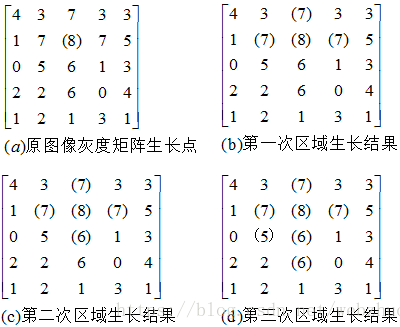示例代码：

#include <opencv2/opencv.hpp> //头文件
#include <opencv2/highgui.hpp>
#include <iostream>
#include <map>

using namespace cv;
using namespace std;

/***************************************************************************************
Function:  区域生长算法
Input:     src 待处理原图像 pt 初始生长点 th 生长的阈值条件
Output:    肺实质的所在的区域 实质区是白色，其他区域是黑色
Description: 生长结果区域标记为白色(255),背景色为黑色(0)
Return:    NULL
Others:    NULL
***************************************************************************************/
void RegionGrow(cv::Mat& src, cv::Mat& matDst, cv::Point2i pt, int th = 40)
{
cv::Point2i ptGrowing;						//待生长点位置
int nGrowLable = 0;								//标记是否生长过
int nSrcValue = 0;								//生长起点灰度值
int nCurValue = 0;								//当前生长点灰度值
matDst = cv::Mat::zeros(src.size(), CV_8UC1);	//创建一个空白区域，填充为黑色
//生长方向顺序数据
int DIR = { { -1, -1 }, { 0, -1 }, { 1, -1 }, { 1, 0 }, { 1, 1 }, { 0, 1 }, { -1, 1 }, { -1, 0 } };
std::vector<cv::Point2i> vcGrowPt;						//生长点栈
vcGrowPt.push_back(pt);							//将生长点压入栈中
matDst.at<uchar>(pt.y, pt.x) = 255;				//标记生长点
nSrcValue = src.at<uchar>(pt.y, pt.x);			//记录生长点的灰度值

while (!vcGrowPt.empty())						//生长栈不为空则生长
{
pt = vcGrowPt.back();						//取出一个生长点
vcGrowPt.pop_back();

//分别对八个方向上的点进行生长
for (int i = 0; i < 8; ++i)
{
ptGrowing.x = pt.x + DIR[i];
ptGrowing.y = pt.y + DIR[i];
//检查是否是边缘点
if (ptGrowing.x < 0 || ptGrowing.y < 0 || ptGrowing.x >(src.cols - 1) || (ptGrowing.y > src.rows - 1))
continue;

nGrowLable = matDst.at<uchar>(ptGrowing.y, ptGrowing.x);		//当前待生长点的灰度值

if (nGrowLable == 0)					//如果标记点还没有被生长
{
nCurValue = src.at<uchar>(ptGrowing.y, ptGrowing.x);
if (abs(nSrcValue - nCurValue) < th)					//在阈值范围内则生长
{
matDst.at<uchar>(ptGrowing.y, ptGrowing.x) = 255;		//标记为白色
vcGrowPt.push_back(ptGrowing);					//将下一个生长点压入栈中
}
}
}
}

}

void on_MouseHandle(int event, int x, int y, int flags, void* param)
{
cv::Mat& src = *(cv::Mat*) param;
cv::Mat src_gray, dst;
if (src.channels() > 1)
cv::cvtColor(src, src_gray, CV_RGB2GRAY);
else
src_gray = src.clone();
cv::Point2i  pt;
switch (event)
{
//左键按下
case cv::EVENT_LBUTTONDOWN:
{
//x:列 y:行
pt = cv::Point2i(x, y);
std::cout << "(x,y)=" << "(" << x << "," << y << ")" << std::endl;
}
break;
//左键放开
char str;
case cv::EVENT_LBUTTONUP:
{
//cv::circle(src, cv::Point2i(x, y), 1, cv::Scalar(0, 0, 255), -1, CV_AA);
//sprintf_s(str, "(%d,%d)", x, y);
//cv::putText(src, str, cv::Point2i(x, y), 3, 1, cv::Scalar(150, 200,0), 2, 8);

pt = cv::Point2i(x, y);
RegionGrow(src_gray, dst, pt);  //区域生长
cv::bitwise_and(src_gray, dst, dst); //与运算
//imshow("src", src);
imshow("dst", dst);
}
break;
}

}

int main()
{
if (src.empty())
{
return -1;
}
/*
int d: 表示在过滤过程中每个像素邻域的直径范围。如果这个值是非正数，则函数会从第五个参数sigmaSpace计算该值。
double sigmaColor: 颜色空间过滤器的sigma值，这个参数的值月大，表明该像素邻域内有越宽广的颜色会被混合到一起，产生较大的半相等颜色区域。
（这个参数可以理解为值域核w_r的\sigma_r）
double sigmaSpace: 坐标空间中滤波器的sigma值，如果该值较大，则意味着越远的像素将相互影响，从而使更大的区域中足够相似的颜色获取相同的颜色。
当d>0时，d指定了邻域大小且与sigmaSpace无关，否则d正比于sigmaSpace. （这个参数可以理解为空间域核w_d的\sigma_d）
int borderType=BORDER_DEFAULT: 用于推断图像外部像素的某种边界模式，有默认值BORDER_DEFAULT.
*/
int d = 7;
double sigmaColor = 140;
double sigmaSpace = 140;

//cv::imshow("原图", src);

cv::Mat gauss_src;
cv::bilateralFilter(src, gauss_src, d, sigmaColor, sigmaSpace, cv::BORDER_DEFAULT);
cv::namedWindow("双边模糊", CV_WND_PROP_AUTOSIZE);//定义一个img窗口
cv::namedWindow("dst", CV_WND_PROP_AUTOSIZE);//定义一个dst窗口
imshow("双边模糊", gauss_src);
cv::setMouseCallback("双边模糊", on_MouseHandle, (void*)&gauss_src);//调用回调函数
cv::waitKey(0);
}



结果：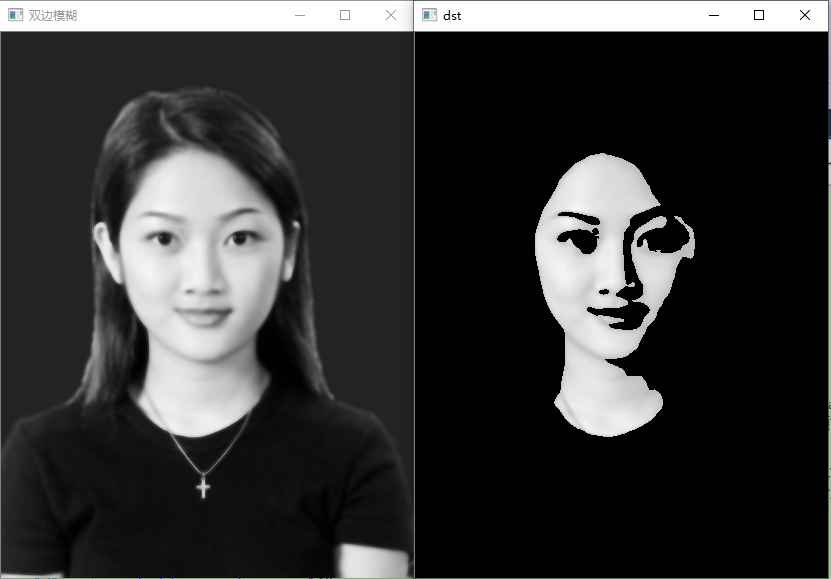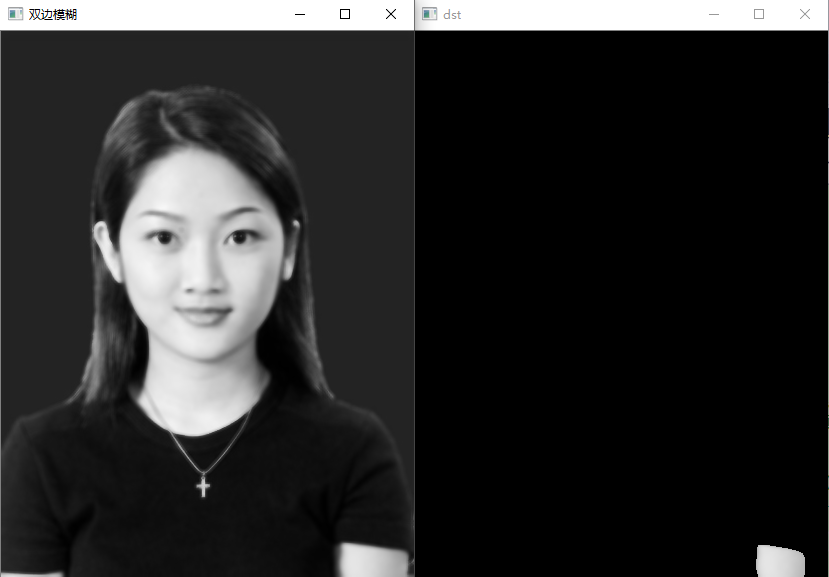展开全文opencv
• 基于Matlab平台的区域生长法图像分割。采用8连通的领域，手动选取种子点。
• 区域生长法: 通俗的讲就是利用初始种子点，通过邻域判断，获取更多的种子点，以达到生长的目的。 有点像是核聚变的链式反应，一个点找到更多的种子点，然后新的种子点再找到更多的，最后生长结束，种子点库也就...

区域生长法:

通俗的讲就是利用初始种子点，通过邻域判断，获取更多的种子点，以达到生长的目的。

有点像是核聚变的链式反应，一个点找到更多的种子点，然后新的种子点再找到更多的，最后生长结束，种子点库也就清空了。

目前主要使用的是四领域和八领域：

四邻域 (左) 和八邻域 (右) 的示意图：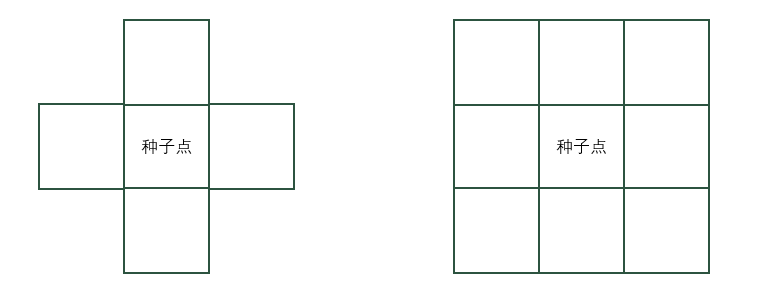区域生长的流程图：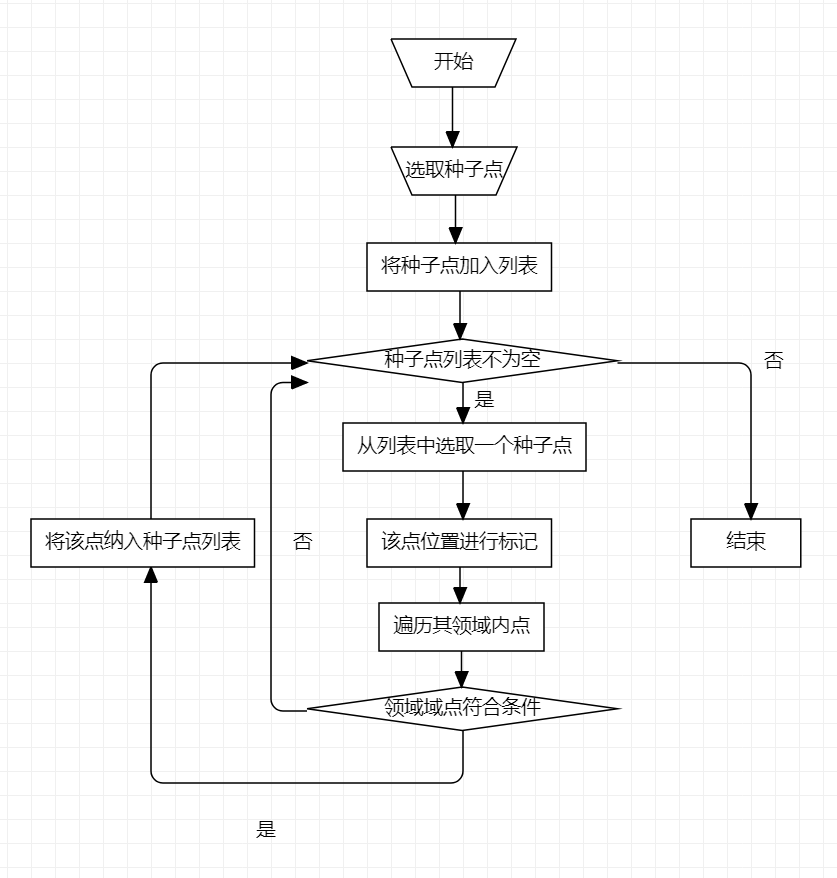以下是部分代码(参考：结合python与遥感图像的区域生长算法实现 - 知乎)：

class Point(object):
def __init__(self, x, y):
self.x = x
self.y = y

def getX(self):
return self.x

def getY(self):
return self.y

def toString(self):
return '[' + str(self.x) + ',' + str(self.y) + ']'

def selectConnects(p):
if p != 0:
connects = [Point(-1, -1), Point(0, -1), Point(1, -1), Point(1, 0), Point(1, 1),
Point(0, 1), Point(-1, 1), Point(-1, 0)]
else:
connects = [Point(0, -1), Point(1, 0), Point(0, 1), Point(-1, 0)]
return connects

def getGrayDiff(img, currentPoint, tmpPoint):
return abs(int(img[currentPoint.x, currentPoint.y]) - int(img[tmpPoint.x, tmpPoint.y]))

def regionGrow(img, seeds, thresh, p=1):
height, weight = img.shape
seedMark = np.zeros(img.shape)
seedList = []
for seed in seeds:
if seed is not None:
seedList.append(seed)
label1 = 255
connects = selectConnects(p)
while len(seedList) > 0:
currentPoint = seedList.pop(0)
seedMark[currentPoint.y, currentPoint.x] = label1
for i in range(0, 8):
tmpX = currentPoint.x + connects[i].x
tmpY = currentPoint.y + connects[i].y

if tmpX < 0 or tmpY < 0 or tmpX >= height or tmpY >= weight:
continue
grayDiff = getGrayDiff(img, Point(currentPoint.y, currentPoint.x), Point(tmpY, tmpX))
if grayDiff < thresh and seedMark[tmpY, tmpX] == 0:
seedMark[tmpY, tmpX] = label1
seedList.append(Point(tmpX, tmpY))
return seedMark

def on_EVENT_LBUTTONDOWN(event, x, y, flags, param):
global num
if event == cv2.EVENT_LBUTTONDOWN:
if num < 5:
num = num + 1
seeds[num] = Point(x, y)
else:
print("已勾选五个点")

结合以上代码和流程图进行分析：

这是一个灰度值图像的区域生长，通过鼠标左键选取五个种子点，在seeds这个数组中我们传入初始的种子点。假设我们从中拿出一个种子点，将其对应位置设置为白色，以这个种子点作为中心实行八邻域生长。判断周围点的灰度值与它的误差是否小于thresh，如果满足条件我们将这个点加入种子点列表，在种子点列表为空时结束循环。

总结：

写这篇文章的目的主要是记录学习内容，代码部分不知道是不是自己的操作问题，但就运行结果来讲原知乎上代码存在坐标颠倒的问题，坐标（x，y）的 x 与 y 应当对于数组 a[ i , j ] 的 j 和 i ，具体表现为选取左下的点，实际却是以右上对称点进行生长。在这里我进行了修改，所以部分代码显示为x，y部分却是y，x。

后续我会基于这个方法对一些医学图像进行一些切割，来获取深度学习的样本，这里做一些进度的记录和学习内容的笔记。如果有什么想法或是问题，欢迎一起讨论。

展开全文python 图像处理
• 江苏科技大学数字图处理之图像分割之区域生长法，本人自己整理的读书笔记
• 区域生长法源代码，在MATLAB开发环境中应用-Region growing method source code, in the MATLAB development environment application...